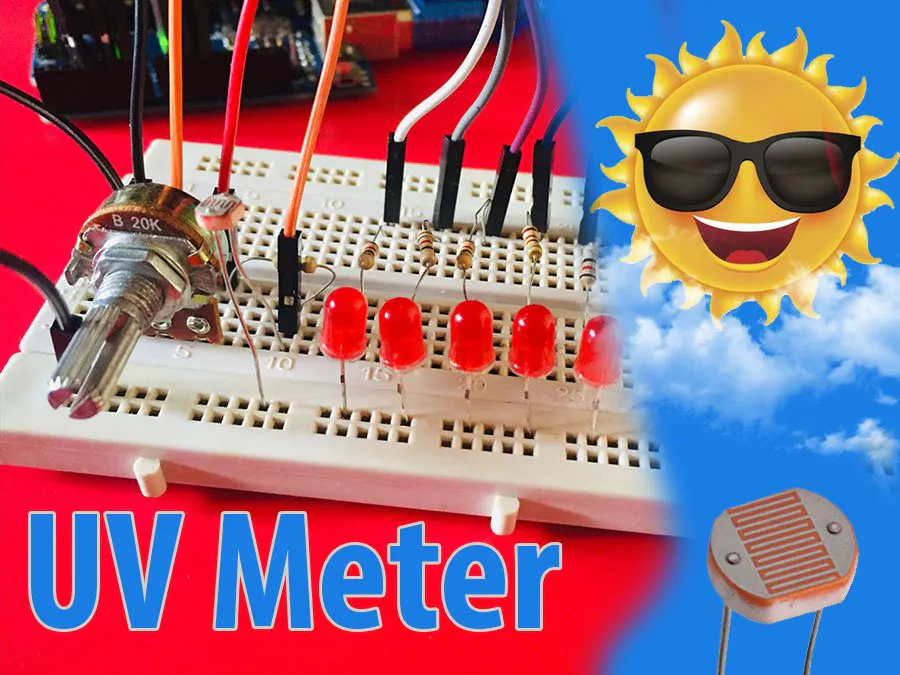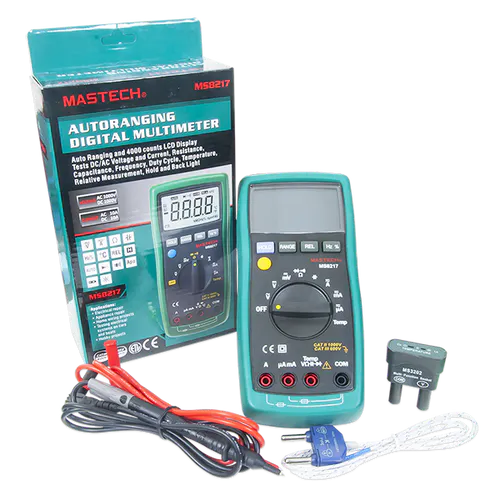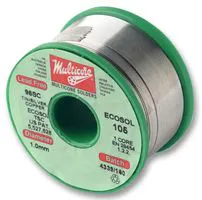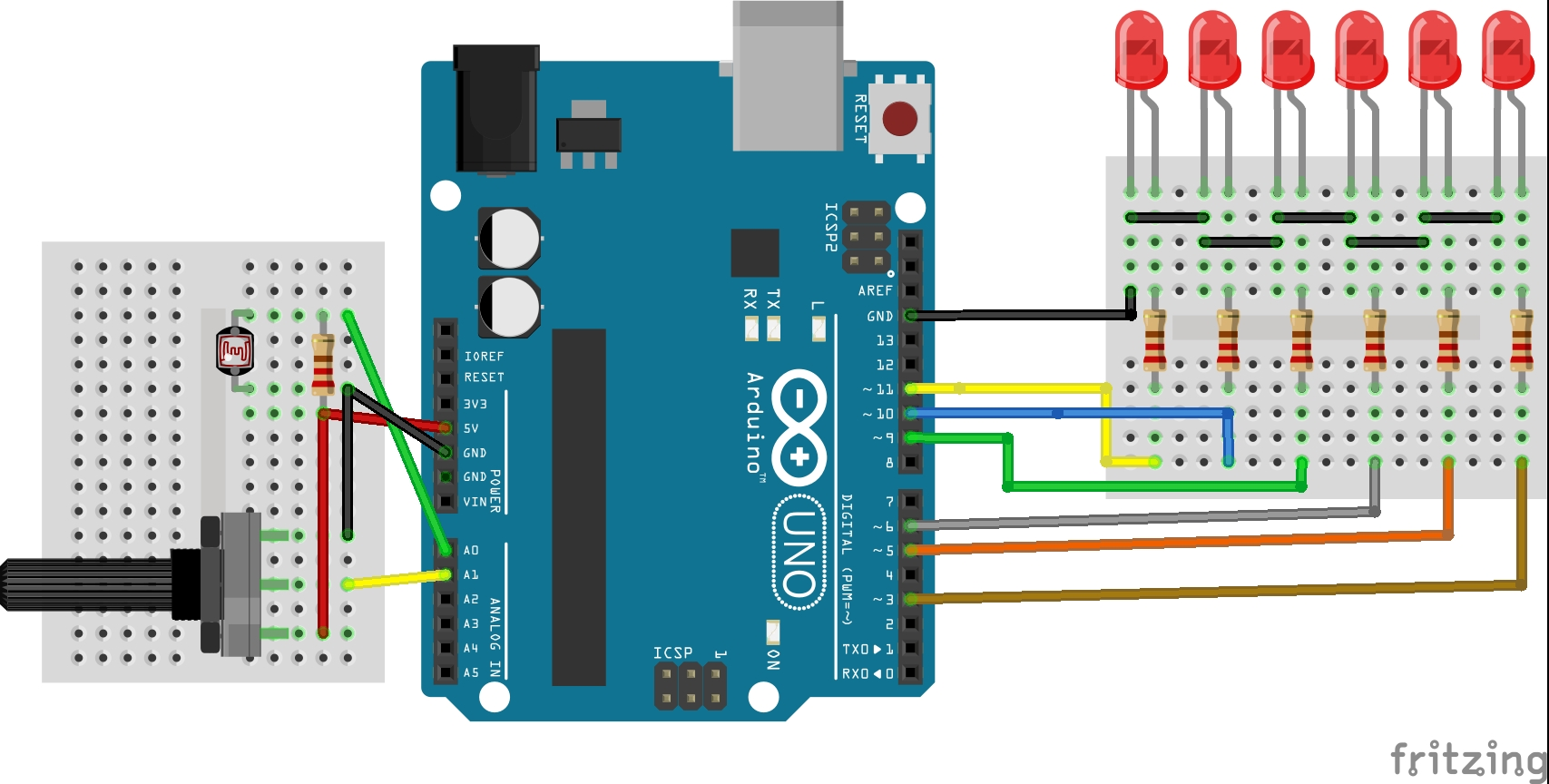Project tutorial# Arduino UV Meter with 1530 Levels © LGPL

LED indicators to show Dark or Light Level

• 2 views
• 0 respects

## Necessary tools and machinesDigilent Mastech MS8217 Autorange Digital MultimeterSoldering iron (generic)This project teach you how to make a Dark/Light level meter with arduino uno.

The project is mainly based on a LDR. When the light comes to the face of the LDR, the resistance of the same is changed. Then the A0 analog pin read the level of the resistance.

Meanwhile you can understand the reason why we have used only 6 LEDs. Because the arduino has only 6 PWM pins. If you want to change the brightness of a LED, you have to use only PWM pins.

As you aware the pin number 3, 5, 6, 9, 10, 11 are the PWM pins.

Addition, I have used one 10k potentiometer to change the sensitivity of the light.

The circuit Diagram is as follows

The wiring part is simple. Even you are a beginner to arduino, you can easily make this project as the code are too simplified for the beginners.

If you watch the following video, you may understand well about the building of this project.

## Code

##### ARduino CodeArduino
```/*
* Programmed by : BMIAK Basnayaka
* Name : Arduino UV Level Meter with 1530 levels
* Date : 09th May, 2020
* email : bmiakbasnayaka@gmail.com
* Blogspot  :https://setnfix.blogspot.com
* Website : http://www.setnfix.com
* Arduino : https://create.arduino.cc/projecthub/SetNFix
* Hackster : https://www.hackster.io/SetNFix
*/

// Pins of 6 LEDs
int led1 = 3;
int led2 = 5;
int led3 = 6;
int led4 = 9;
int led5 = 10;
int led6 = 11;

int ldr = A0;//LDR sensor pin
int sen = A1;//10k Potentiometer

//All the bulbs are off at the beginning
int a=0;
int b=0;
int c=0;
int d=0;
int e=0;
int f=0;

int bright = 0; // Analog level is 0 (LED off 0 -255 on)
int ldrlevel = 0; // Analog read of LDR
int senlevel = 0;// Analog Read of potentiometer

void setup() {
pinMode(led1,OUTPUT);
pinMode(led2,OUTPUT);
pinMode(led3,OUTPUT);
pinMode(led4,OUTPUT);
pinMode(led5,OUTPUT);
pinMode(led6,OUTPUT);
pinMode(ldr,INPUT);
pinMode(sen,INPUT);
Serial.begin(9600);

}

void loop() {

//delay(100);

bright = map(ldrlevel,senlevel,1023,0,1530);

//if (bright<0) bright =0;
Serial.println(bright);
a = bright;
b = bright - 255;
c = bright - 510;
d = bright - 765;
e = bright - 1020;
f = bright - 1275;

if (a >255) a=255;
if (b >255) b=255;
if (c >255) c=255;
if (d >255) d=255;
if (e >255) e=255;
if (f >255) f=255;

if (a < 0) a=0;
if (b < 0) b=0;
if (c < 0) c=0;
if (d < 0) d=0;
if (e < 0) e=0;
if (f < 0) f=0;

analogWrite(led1,a);
analogWrite(led2,b);
analogWrite(led3,c);
analogWrite(led4,d);
analogWrite(led5,e);
analogWrite(led6,f);

}
```

## Schematics

Circuit DiagramMay 9, 2020

#### Members who respect this project

See similar projects
you might like

#### Arduino RTC LED Clock

Project tutorial by SetNFix

• 5,199 views
• 14 respects

#### DIY UV Meter With Arduino and a Nokia 5110 Display

Project tutorial by Nick Koumaris

• 3,130 views
• 1 comment
• 12 respects

#### A UV Measurer for Umbrellas

Project showcase by NTPC Young Maker Studio

• 1,905 views
• 1 comment
• 7 respects

#### Simple Vu Meter Using Arduino

Project tutorial by edison science corner

• 116 views
• 0 respects

#### Water Level Meter

Project showcase by Fanatic Series

• 22,651 views